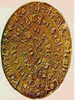classical cryptography

• 古典密码算法

2018-10-08 17:08:39
通过编程实现替代密码算法和置换...实验内容：古典密码算法曾被广泛应用，大都比较简单。它的主要应用对象是文字信息，利用密码算法实现文字信息的加密和解密。其中替代密码和置换密码是具有代表性的两种古典密码算法。
• 使用java编写的古典密码算法（替代密码算法和置换密码算法）
• 基于古典密码学的密码器设计，陈焰，，本文基于古典密码学的维吉尼亚密码和列位密码，配合异或运算设计了一个简单实用的密码器。该密码器对于ASCII码表内的字符经过三重�维吉尼亚密码
• 四种古典密码的c++算法实现，有playfair密码，vegenere密码，置换密码，移位密码。包含四种密码的加密和解密源代码以及可执行文件，代码注释清晰。
•古典密码
• 实现维吉尼亚密码，用键盘接收明文和密钥，屏幕打印密文和解密后的明文。用维吉尼亚密码实现控制台对英文文本文件（注意明文和密文都以文件形式存在）的加解密 形式：cipher -e/-d key inputfile outputfile
• 加密:把明文中每个字母替代为字母表中其后的第k个字母。解密:与加密相反，把密文中每个字母替代为字母表中其前的第k个字母。
•python
• 自己写的密码学报告，里面有完整的实验目的、流程图、关键代码分析、代码、运行截图等。古典密码主要的两个程序——置换密码、代换密码，写的超级清晰！欢迎下载
• 简单的凯撒密码，希尔密码加解密，python实现
• 古典密码算法的设计与实现（C++实现） ，仿射密码，置换密码，hill密码，序列密码https://blog.csdn.net/qq_32261191/article/details/78802233古典密码算法
•古典密码
• 古典密码实验

2015-07-01 01:05:23
古典密码算法实验报告，密码学实验，计算机网络安全实验古典密码
• 该压缩包包含了Python脚本编写的摩斯密码加密解密、培根密码加密解密、ASCII编码解码、凯撒密码加密解密，可用于教学和实操
• 古典密码

万次阅读 2019-05-16 17:50:38
密码学的阶段划分 ... 对称密码阶段（1949-1975年） 在这之后就进入到了现代密码学的阶段，和古典密码阶段的主要区别在于这个阶段的加密和解密算法无需保密，信息的安全性主要依赖于对秘钥的保密。需要解...

密码学的阶段划分

密码学的发展按照其对算法和秘钥的保密程度大致可以分为如下三个阶段。

• 古典密码阶段（1949年前） 在这个阶段算法和秘钥都是保密的，秘钥空间较小，信息的安全性主要依赖于对于加密和解密算法的保密。
• 对称密码阶段（1949-1975年） 在这之后就进入到了现代密码学的阶段，和古典密码阶段的主要区别在于这个阶段的加密和解密算法无需保密，信息的安全性主要依赖于对秘钥的保密。需要解决的主要问题是在不可信信道下的秘钥传输问题。
• 公钥密码阶段（1976年-至今） 在公钥密码阶段，加密秘钥（公钥）可以公开，仅对解密秘钥（私钥）保密，基于一些数学难题保证很难通过公钥推出私钥。

数学知识

• 整除与约简
• 同余
• 素数的相关性质 每一个正整数都可以分解为一系列素数的乘积，且这种分解是唯一的。

n=pe11+pe22...pemmei>0,i=1,2,....mn=p1e1+p2e2...pmemei>0,i=1,2,....m

• 欧几里得算法和拓展欧几里得算法 欧几里得算法（辗转相除法）略 在学拓展欧几里得算法的时候遇到了一些困难就多记一下了。 先说一条定理：对于任意x和y不同时为0的整数，x和y的最大公因子（x，y）是以ax+byax+by表示的最小整数。 而拓展欧几里得算法就是用来求这个a和b的。下图是计算方法的推导过程：通过上面的推导可以写出下面这样的一个式子：

[011−qn][011−qn−1]...[011−q1][xy]=[gcd(x,y)0][011−qn][011−qn−1]...[011−q1][xy]=[gcd(x,y)0]

将矩阵相乘即可求得a，b，根据求解方向的不同可以得到递归和非递归的算法，矩阵从左向右算为递归算法（从左向右也可以写成非递归算法，不过需要一个数组记录qnqn），矩阵从右向左算法为非递归算法。 递归算法实现：

void exgcd(int a,int b,int &x,int &y)
{
if(b==0)
{
x=1;
y=0;
return;
}
exgcd(b,a%b,x,y);
int t=x;
x=y;
y=t-a/b*y;
return;
}

非递归算法一实现

void exgcd(int m,int n,int &x,int &y)
{
int r=1;
vector<int> qList;
while (r!=0){          //循环计算q并放入vector中         r=m%n;
int q=m/n;
qList.push_back(q);
m=n;
n=r;
}
int listSize=qList.size();
x=0;
y=1;
for(int i=listSize-2;i>=0;--i){ //计算x，y         int tempX=x;
x=y;
y=tempX-y*qList[i];
}
}

非递归算法二实现：

void exgcd(int m,int n,int &x,int &y)
{
int x1,y1,x0,y0;
x0=1; y0=0;
x1=0; y1=1;
x=0; y=1;
int r=m%n;
int q=(m-r)/n;
while(r)
{
x=x0-q*x1; y=y0-q*y1;
x0=x1; y0=y1;
x1=x; y1=y;
m=n; n=r; r=m%n;
q=(m-r)/n;
}
}

• 乘法逆元 定义：n模m的乘法逆t记做（n−1%mn−1%m）满足nt%m=1
乘法逆存在的条件：（n，m）=1
简单证明：
n
t%m=1 =>nt=km+1
(km+1,km)=1 => (km+1,m)=1 =>(nt,m)=1
(n*t,m)=1 =>(n,m)=1
得证
计算乘法逆的方法：使用拓展欧几里得算法求得an+bm=1,则a为其乘法逆。

古典密码学-代替密码

密码学中对信息进行处理的主要方式有两种换位和代替，顾名思义换位就是将原有的明文字符的顺序打乱，而代替则是按照一定的规律将明文字符替换成一些其他的字符。按照处理方式的不同，具体的分类如下图所示（盗的上课ppt上的图(◔◡◔)）：下面就分别介绍一些常见的古典密码的实现。

Caesar 密码

Caesar 密码非常简单，它的一般表达式如下所示：

y=f(x)=(x+k)%26（加密）x=f(y)=(y−k)%26（解密）y=f(x)=(x+k)%26（加密）x=f(y)=(y−k)%26（解密）

Caesar密码的加密秘钥k和解密秘钥是相同的，同时秘钥空间的大小为25，通过不断尝试k的数值我们可以非常轻易的得到秘钥。

仿射密码

由于Caesar 密码的秘钥空间较小，通过增加参数提升秘钥空间大小就得到了仿射密码。

y=Ea,b(x)=(ax+b)%26（加密）　　y=Da,b=(a−1y−a−1b)%26（解密）y=Ea,b(x)=(ax+b)%26（加密）　　y=Da,b=(a−1y−a−1b)%26（解密）

通过分析我们可以知道其秘钥空间大小为311，其计算公式为12*26-1。12表示的是a的可能性，因为a模26的乘法逆必须存在，所以其值为φ(26)，26为b的可能性，减一是a=1，b=0的特殊情况。仿射密码的秘钥长度虽然得到扩大但是依然有限，同时其本质上依然为单表代替密码，密文中依然保留着明文中字符的统计规律，易被破解。

Vignere密码

Vignere的多表代替密码中最著名的也是最简单，它本质上不过是多个Caesar密码组合，每隔一位就换一个Caesar密码的秘钥，直到结束又开始循环，其秘钥序列表示为：K=k0,K1,K2,…Km−1K=k0,K1,K2,…Km−1，可以看出Caesar密码是Vignere密码秘钥长度为1时的特殊情况，具体加解密公式如下：

yi=(xi+ki%m)%26（加密）xi=(yi−ki%m)%26（解密）yi=(xi+ki%m)%26（加密）xi=(yi−ki%m)%26（解密）

可以看出Vignere密码的秘钥空间为26m26m,秘钥空间可以说是非常大了，但是因为其还是保留许多频率分布的特征，通过Kasiski测试法和重合指数攻击在秘钥不大长的情况下可以比较简单的进行破解，对多表替代密码的攻击方式下次再写。

OTP密码（一次一密密码）

算法原理：加密的秘钥和明文一样长，而且秘钥本身只使用一次。具体的加密方式可以任意可以是Vignere密码也可以是Vernam密码。
一次一密密码在理论上保证了信息的完全安全，因为任意一段有意义的明文都会对于一段唯一的秘钥，而攻击者如果采用穷举攻击的方式，将会得到大量有意义的明文，攻击者将无法判断哪个才是正确的。缺点：大规模随机秘钥的产生非常困难，同时更为麻烦的是秘钥的分发和保存。

古典密码-换位密码

换位密码顾名思义就是不改变明文中的字母仅改变明文中字母的次序，常见的方式有列移位加密，具体方式如下图所示：解密方式和加密方式类似。

展开全文• 本文作为学习笔记来使用，对正在上密码学课程的学生来说例题比较有价值，此外在图片和文字上大量参考了各种密码学书籍，并添加了自己的注解和理解；

本文作为一篇学习笔记，在图片和文字上大量参考了各种密码学书籍，并添加了自己的注解和理解；古典密码学的学习需要一些数论的知识，比如模运算、扩展的欧几里得算法；在入门学习过程中没必要系统地学习数论，只需学习必要知识即可

古典密码的加密思想和方法在现代密码学中仍然在使用，古典密码的加密技术主要分为

• 替代技术
• 置换技术

参考资料

《网络安全理论与应用》余研 付安民 …编著
《图解密码技术》第三版 结成浩著

替代技术

替代技术是将明文中的每个元素映射为另一个元素的技术。若把明文视为二进制序列，则替代技术就是用密文序列来代替明文序列。代替后，字母位置不变，其本身的值发生改变。而后面介绍的置换技术是字母的值不变，字母在明文中的位置发生改变。

注解：
DES的S盒就是一张替代表
元素指的是：字母、比特、比特组合或字母组合

根据替代是对每个字母逐个进行还是对多个字母同时进行，古典密码又可以分为

• 单字母表替代密码
• 多字母表替代密码

基于替代技术的古典密码有凯撒密码、移位密码、仿射密码、Vigenere密码、Hill密码等。

单字母表替代密码

凯撒密码

凯撒密码是已知最早的替代密码。凯撒密码将字母表视为一个循环的表，将明文中的每个字母使用字母表中其后的第三个字母来代替；
凯撒密码中明文字母和密文字母的对应关系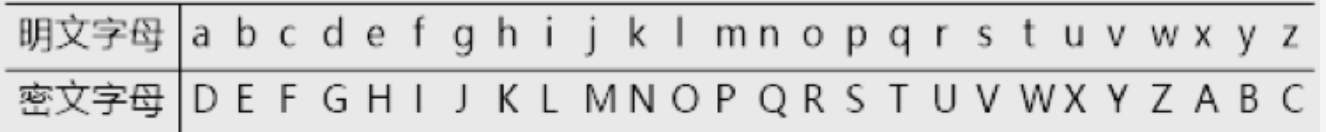例如
若明文为i will go fishing tomorrow
则密文为L ZLOO JLVKLQJ WRPRUURZ

若令每个字母对应一个数字（a=0, b=1, …, z=25），m表示明文字母，c表示密文字母，且0≤m,c≤25，则凯撒算法的加密和解密可以形式化表示为
c = E 3 ( m ) = ( m + 3 ) m o d 26 c=E_3 (m)=(m+3) mod 26
m = D 3 ( c ) = ( c − 3 ) m o d 26 m=D_3 (c)=(c-3) mod 26
可见，3是加解密所使用的密钥。
在凯撒密码中，密钥的值固定为3，因此很容易对凯撒密码进行攻击。

移位密码

为了增大凯撒密码的密钥空间，将凯撒密码的加解密算法进行一般化，定义密文字母和明文字母的偏移可以是任意值，即定义移位密码的加密和解密形式为
c = E k ( m ) = ( m + k ) m o d 26 c=E_k (m)=(m+k) mod 26
m = D k ( c ) = ( c − k ) m o d 26 m=D_k (c)=(c-k) mod 26
其中，0≤m,c≤25，k为算法的密钥，且0≤k≤25。

尽管移位算法将密钥空间扩展至字母表的长度，但其密钥空间仍然太小，只有26种可能的取值，仍然可以轻易地穷举整个密钥空间来破译密文，平均只需尝试26/2=13次即可计算得到明文。

仿射密码

移位密码的密钥空间太小，仿射密码就是移位密码的更一般形式；在仿射密码中，加密函数定义为
c = E ( a , b ) ( m ) = ( a m + b ) m o d 26 c=E_{(a,b)} (m)=(am+b) mod 26 (1)
其中 a , b ∈ Z 26 a,b∈Z_{26} 。形如上式的函数被称为仿射函数，因此该密码体制也被称为仿射密码
特别地，当a = 1时，移位密码为仿射密码的特殊形式。

为了能对密文进行解密，必须保证所使用的仿射函数是一个单射函数，即对于任意的 c ∈ Z 26 c∈Z_{26} ，同余方程 a m ≡ ( c − b ) m o d 26 am≡(c-b) mod 26 有惟一解m

可以证明，当 g c d ( a , 26 ) = 1 gcd(a,26)=1 时， a a Z 26 Z_{26} 上存在乘法逆元 a − 1 a^{-1} ，使得同余方程存在惟一解： m = a − 1 ( c − b ) m o d 26 m=a^{-1} (c-b) mod 26 (2)

(2)式由同余的除法得到
gcd是greatest common digital, 其中gcd(c,m)表示c和m的最大公约数

因此，仿射密码的解密函数可以定义为
m = D ( a , b ) ( c ) = a − 1 ( c − b ) m o d 26 m=D_{(a,b)} (c)=a^{-1} (c-b) mod 26
其中，a,b是密钥，且为满足 ( a , b ) ∈ Z 26 × Z 26 (a,b)∈Z_{26}×Z_{26} g c d ( a , 26 ) = 1 gcd(a,26)=1 的整数。

案例
加密：解密：加密很容易计算，这里不在叙述；
解密使用扩展的欧几里得算法：步骤如下

• 用扩展的欧几里得算法求的乘法逆元a-1
• 乘法逆元算出来后，进行模运算即可
求乘法逆元手算如下

因为 7 x = 1 m o d 26 7x = 1mod 26 ， x的取值即为7mod26下的乘法逆元
7 x + 26 y = g c d ( 7 , 26 ) = 1 7x + 26y = gcd(7,26)=1 记为 (2)
用扩展的欧几里得算法求出x即可

运算过程如下：
第一步：进行辗转相除（写成等式形式）

26 = 3×7 + 5； ⇒ 5 = 26 - 3×7
7 = 1×5 + 2； ⇒ 2 = 7 -1×5
5 = 2×2 + 1; 1 = 5 - 2×2

其中 26为被除数，3为商，7为除数，5为余数；将余数5用26和7表示出来，方便后面进行回带

回带过程
为了求出x，根据(2)式用7和26表示1即可算出x和y的值；可以通过保持商不变，替换掉除1以外的所有余数来实现；

1 = 26 - 3×7 - 2×[ 7 - 1×(26 - 3×7 ) ] 这里替换掉上面式子中的余数2和余数5；
1 = 3×26 - 11×7 可得到 x=-11 y=3
x = -11 mod 26 等价于 x = (-11 + 1×26) mod 26 等价于x = 15 mod 26

为了方便计算，这里取x=15即可
因此在计算时，7mod26下的乘法逆元为15；
解密函数为
m = D 7 , 3 ( c ) = 15 ( c − 3 ) m o d 26 m = D_{7,3} (c) = 15(c - 3) mod 26

计算举例
当c=4时，m=15(4- 3)mod 26 = 15

针对单字母表替代密码的攻击

单字母表替代密码可以实现字母表中26个字母的任意替代，密钥空间大小为26!，即有大于 4 × 1 0 26 4×10^{26} 种可能的密钥，即使每微秒尝试一个密钥，也需要花费约 1 0 13 10^{13} 年才能穷举所有的密钥。因此，单字母表替代密码可以抵御强行攻击。

然而，如果密码攻击者知道明文的性质（例如，明文是自然语言文本），则攻击者可以利用该自然语言的某些统计特性实施攻击。

例如，在英语中，字母出现频率依次为e(12.75%)，其次分别为t(9.25%)、r(8.5%)、i(7.75%)、n(7.75%)以及o(7.5%)等，字母的出现频率呈现出一定的统计特性。

攻击者可以根据密文中字母、双字母组合（如th）甚至三字母组合（如the）出现的相对频率进行猜测，只要密文足够长，攻击者即可由明文推导出密文

攻击者利用明文的统计特性针对单字母替代密码实施攻击的步骤如下：

1. 攻击者确定密文中字母出现的相对频率；
2. 将密文字母的出现频率与自然语言的标准频率分布进行比较；
3. 还可以观察双字母组合和三字母组合等，以便对明文进行猜测。

频率分析的具体案例可以参考《图解密码技术》

局限性：由于单字母替代密码反映了原来字母表的频率特性，因此很容易被攻破；

多字母表替代密码

为了减少密文中明文结构的残余度，改善单字母密码的强度，出现了多字母密码技术。
在多字母替代密码中，处理明文消息时使用了不同的单字母替代。

多字母替代密码通常具有以下性质：

• 使用一系列相关的单字母替代规则；
• 由密钥决定对于一个给定的变换选择哪种特定的规则。

典型的多字母表替代密码包括Vigenère密码、Hill密码等。

Vigenere密码

字母表替代密码中最著名和最简单的密码是Vigenère密码，其发明者是16世纪的法国人Blaise de Vigenère
Vigenère密码的替代表由26个移位密码替代表组成，它在处理明文消息中的每个字母时使用了不同的单字母替代。

加密过程可以形式化描述为 c i = ( m i + k i m o d r ) m o d 26 c_i=(m_i+k_{i mod {r}}) mod 26

因此，Vigenère密码本质上是对每个明文字母使用了不同的单字母替代密码，而具体使用哪个单字母替代表则是由密钥字母确定。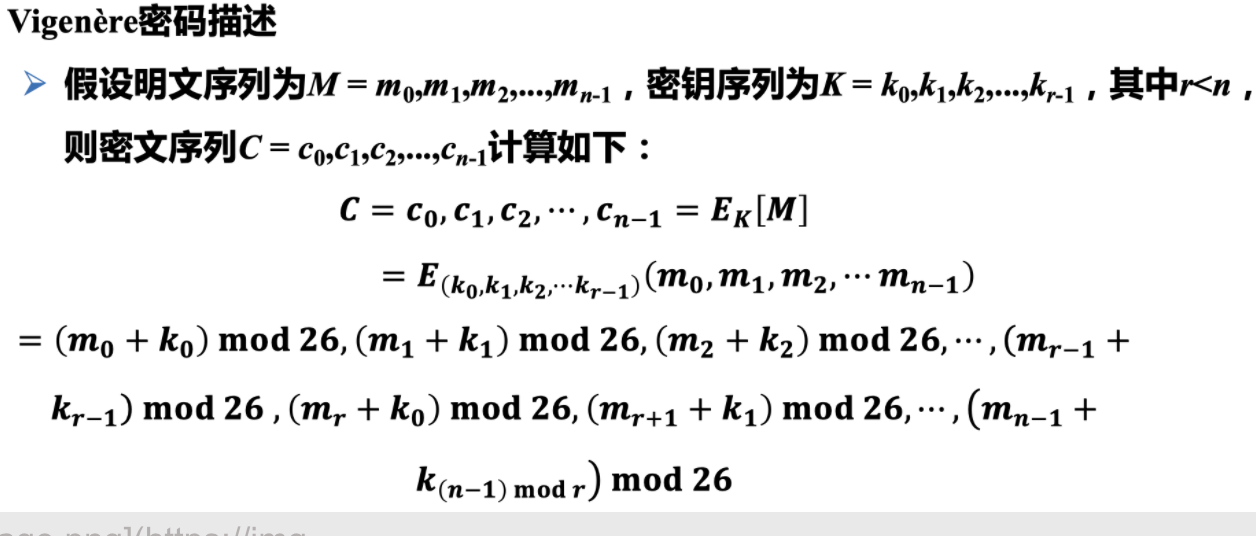解密过程如下：
m i = ( c i − k i m o d r ) m o d 26 m_i=(c_i-k_{i mod r}) mod 26
Vigenère密码的加解密过程中，重复使用相同的密钥字母串K，直至所有的消息均加解密完成。

案例
解：密钥对应的数字串为K=(7,0,15,15,24,19,8,12,4)，将密钥串重复书写在由明文串转换的数字上方，进行模26下的加密运算，如下所示：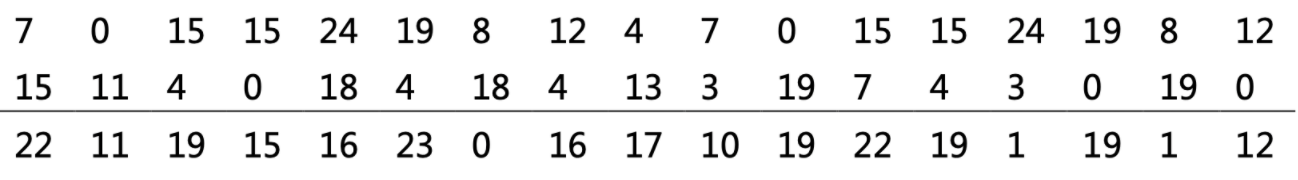对应的密文串为WLTPQXAQRKTWTBTBM
解密时使用相同的密钥字进行逆运算即可。

总结
Vigenère密码中不同的密钥对应着不同的单字母替代，其密钥空间的大小为 2 6 r 26^r ，即使r值很小，使用穷尽搜索方法也需要很长的时间才能遍历密钥空间，因此，多字母表替代密码的安全性要比单字母表替代密码的安全性要好

Hill密码(希尔密码)

Hill密码是一种具有代表性的多字母表替代密码，由Lester S. Hill于1929年提出。
Hill密码使用了线性变换来实现加密解密功能。

加密过程
在Hill密码中，首先将明文M划分为由n个字母构成的分组 M = ( m 1 , m 2 , . . . , m n ) M=(m_1,m_2,...,m_n) ，密钥K取为n×n的矩阵，对于明文M和密钥K，计算密文C如下：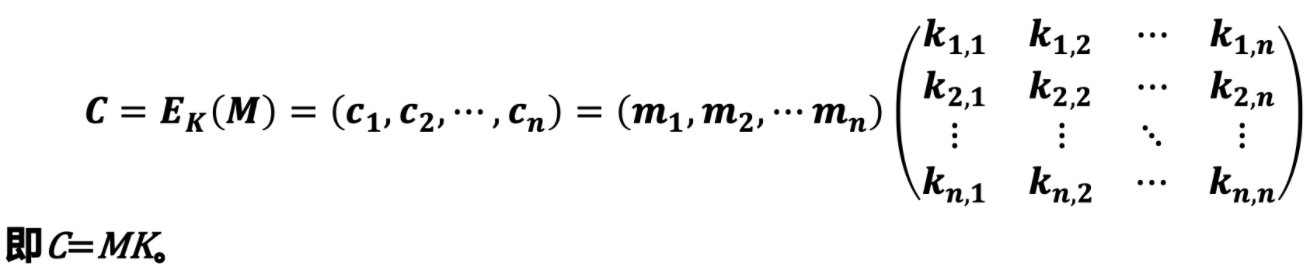解密过程
在Hill密码中密文是通过明文进行线性变换得到。若要从密文中计算得到明文，需要进行解密变换，相应的明文应为 M = C K − 1 M=CK^{-1} 。即要求矩阵 K K 在模 q q 的情形下存在可逆矩阵 K − 1 K^{-1}
由线性代数可知，矩阵K在模q的情形下存在可逆矩阵K-1的充分必要条件是 g c d ( ∣ K ∣ , q ) = 1 gcd(|K|, q)=1 ，且 K − 1 = ∣ K ∣ − 1 K ∗ K-1=|K|-1K^{*}
因此，Hill密码的解密过程为
M = C K − 1 m o d q M=CK^{-1} mod q

置换技术

对于基于替代技术的密码而言，其明文字母被不同的密文字母所代替。而置换密码则不同，置换技术是在不丢失信息的前提下对明文中的元素进行位置上的重新排列，以打乱明文字母的位置和顺序。

在对称密码中，经常使用各种置换表进行置换选择。

定义在有限集X上的一个置换是一个双射函数 f : X → X f:X→X 。即对于任意的 y ∈ X y∈X ，存在唯一的 x ∈ X x∈X 使得 f ( x ) = y f(x)=y ，从而可以定义置换f的逆置换： f − 1 : X → X f^{-1}:X→X
f − 1 ( y ) = x f^{-1} (y)=x 当且仅当 f ( x ) = y f(x)=y
其中， f − 1 f^{-1} 也是X上的一个置换。

置换密码的定义

假设M为明文，C为密文，令n是一正整数 ， x i , y i ∈ Z 26 ，x_i,y_i∈Z_{26} ，K是由所有定义在集合{1,2,…,n}上的置换组成，对于任意的置换（即密钥）f，加密过程为
C = E f ( x 1 , x 2 , ⋯ , x n ) = ( x f ( 1 ) , x f ( 2 ) , ⋯ , x f ( n ) ) C=E_f(x_1,x_2,⋯,x_n )=(x_{f(1)} ,x_{f(2)} ,⋯,x_{f(n)} )
解密过程为
M = D f ( y 1 , y 2 , ⋯ , y n ) = ( x f − 1 ( 1 ) , x f − 1 ( 2 ) , ⋯ , x f − 1 ( n ) ) M=D_f(y_1,y_2,⋯,y_n )=(x_{f^{-1} (1) },x_{f^{-1} (2) },⋯,x_{f^{-1} (n) })
其中， f − 1 f^{-1} 是置换 f f 的逆置换。

案例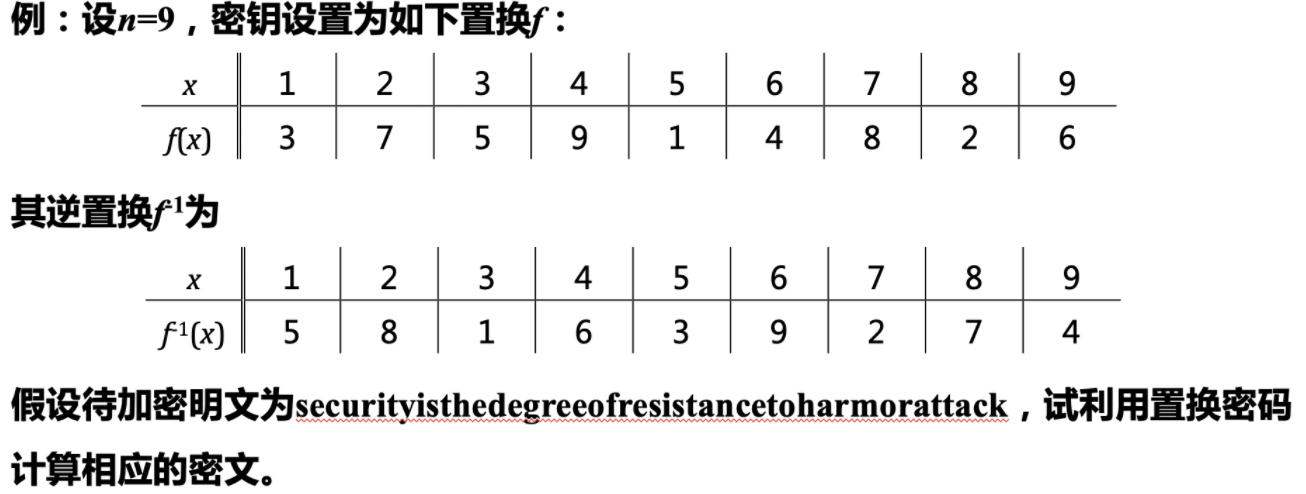解：首先将明文分组，每9个一组：
securityi|sthedegre|eofresist|ancetohar|morattack
对每组的9个字母使用加密变换f，可得密文：
CTRISUYEI|HGDESERTE|FIETERSOS|CHTRAEANO|RATKMACOT
解密过程使用逆置换 f − 1 f^{-1} ，可恢复明文
securityi|sthedegre|eofresist|ancetohar|morattack

展开全文古典密码学
• 和：(9条消息) 古典密码集合_Sunny-CSDN博客_古典密码 23、维吉尼亚密码（Vigenère Cipher） 【Vigenère Cipher】 　由于频率分析法可以有效的破解单表替换密码，法国密码学家维吉尼亚于1586年提出一...

一、密码类型汇总。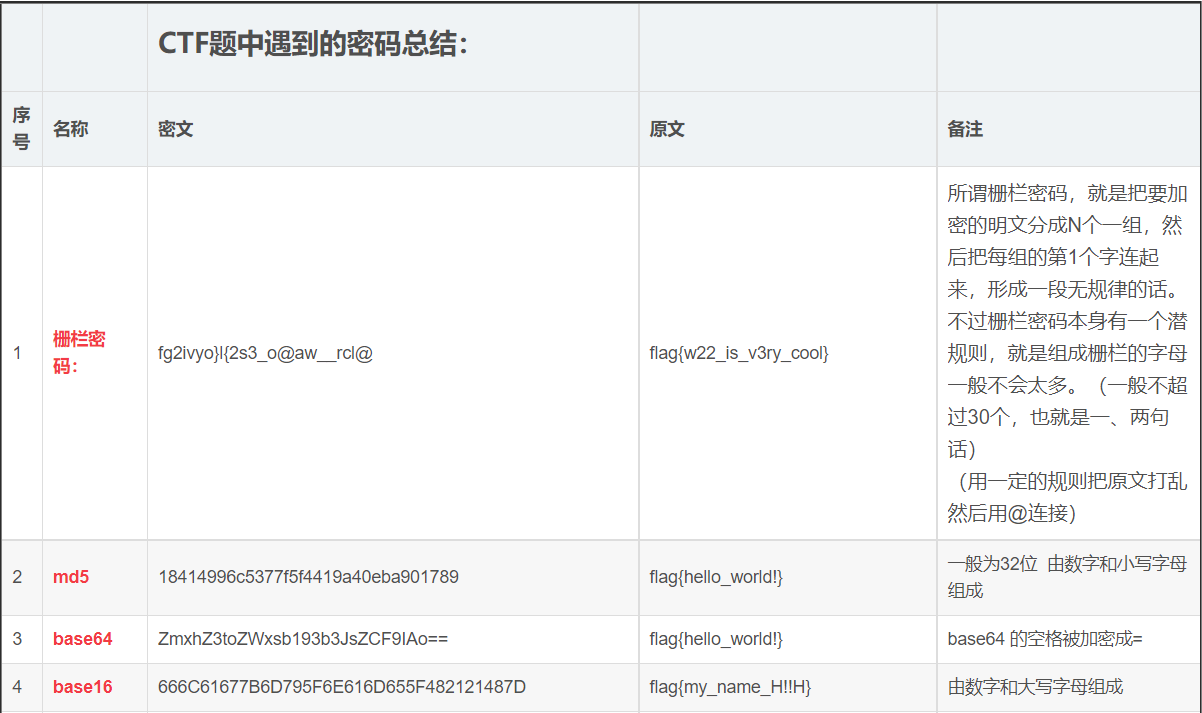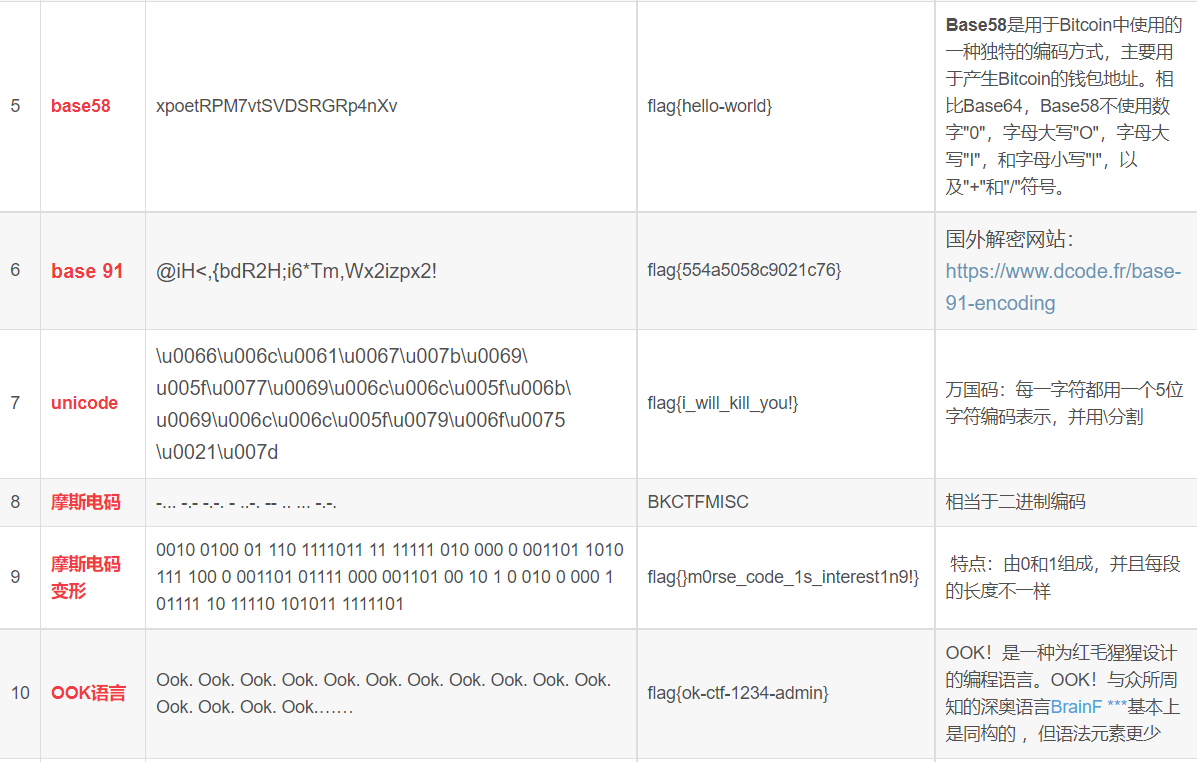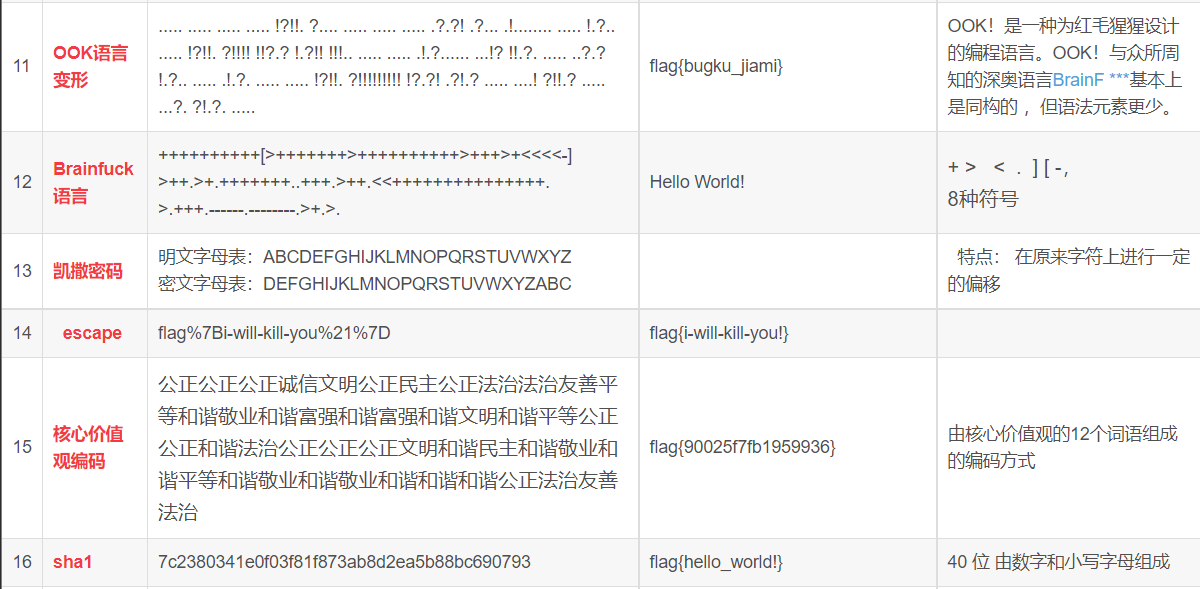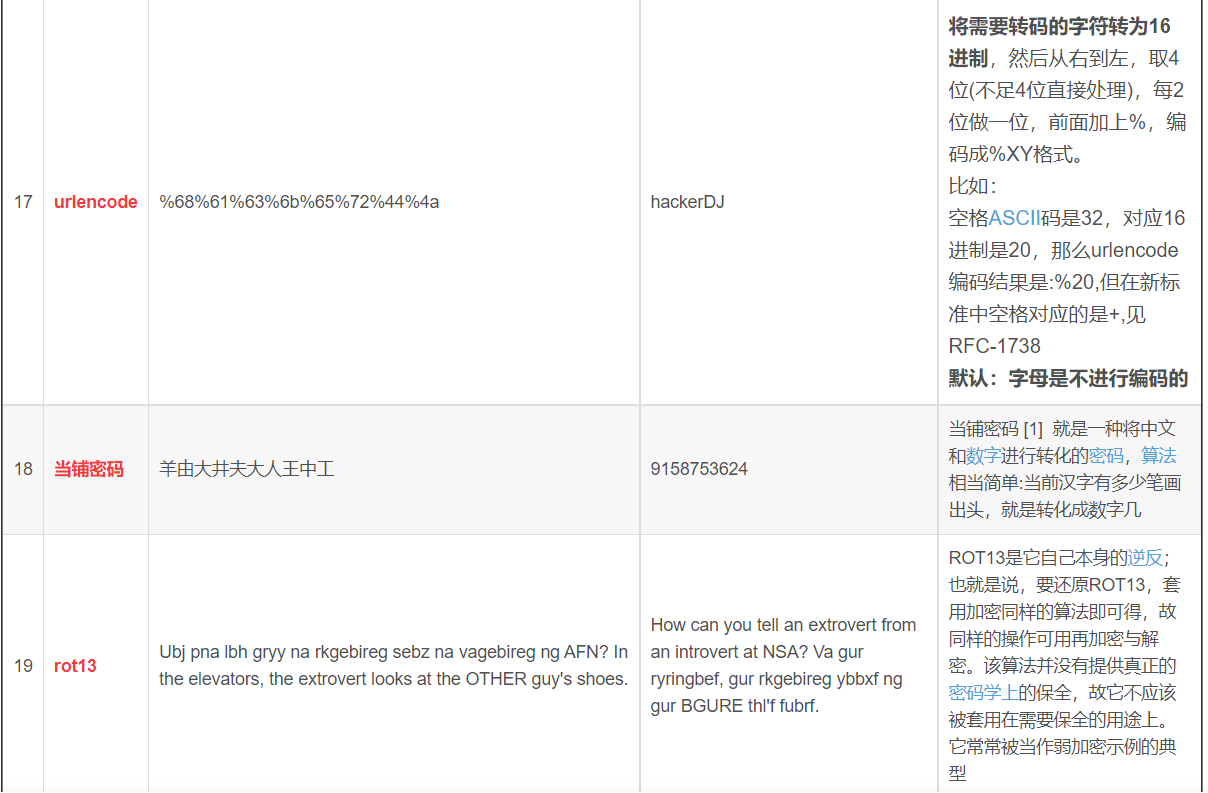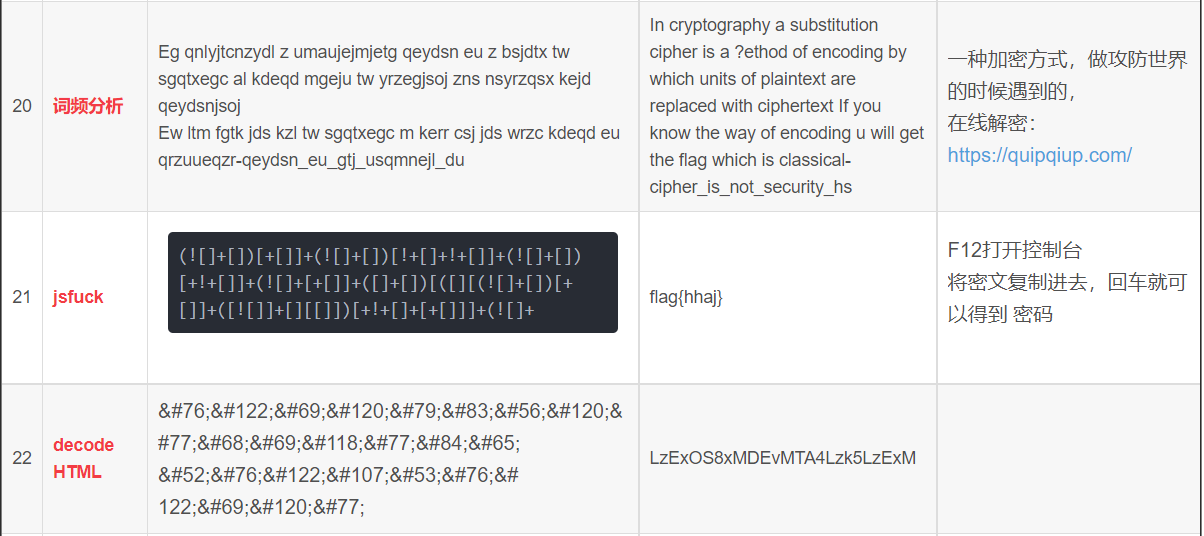23、维吉尼亚密码（Vigenère Cipher）

【Vigenère Cipher】

由于频率分析法可以有效的破解单表替换密码，法国密码学家维吉尼亚于1586年提出一种多表替换密码，
即维吉尼亚密码，也称维热纳尔密码。维吉尼亚密码引入了“密钥”的概念，即根据密钥来决定用哪一行的密表来进行替换，
以此来对抗字频统计。

加密算法：例如密钥的字母为[d]，明文对应的字母[b]。根据字母表的顺序[d]=4,[b]=2，那么密文就是[d]+[b]-1=4+2-1=5=[e]，
因此加密的结果为[e]。解密即做此逆运算。

加密公式：密文 = (明文 + 密钥) Mod 26 - 1
解密公式：明文 = [26 + (密文 - 密钥)] Mod 26 + 1

也可以用查表法来进行加密：例如密钥的字母为[d]，明文对应的字母[b]，在下图的表格第一行找到字母"d"(深蓝色)，
再在左边第一列找到字母"b"(绿色)，两个字母的交叉点(b行d列)就是字母"E"，所以对应的密文字母为[e]。

[-----------------图-----------------]

a b c d e f g h i j k l m n o p q r s t u v w x y z
a A B C D E F G H I J K L M N O P Q R S T U V W X Y Z
b B C D E F G H I J K L M N O P Q R S T U V W X Y Z A
c C D E F G H I J K L M N O P Q R S T U V W X Y Z A B
d D E F G H I J K L M N O P Q R S T U V W X Y Z A B C
e E F G H I J K L M N O P Q R S T U V W X Y Z A B C D
f F G H I J K L M N O P Q R S T U V W X Y Z A B C D E
g G H I J K L M N O P Q R S T U V W X Y Z A B C D E F
h H I J K L M N O P Q R S T U V W X Y Z A B C D E F G
i I J K L M N O P Q R S T U V W X Y Z A B C D E F G H
j J K L M N O P Q R S T U V W X Y Z A B C D E F G H I
k K L M N O P Q R S T U V W X Y Z A B C D E F G H I J
l L M N O P Q R S T U V W X Y Z A B C D E F G H I J K
m M N O P Q R S T U V W X Y Z A B C D E F G H I J K L
n N O P Q R S T U V W X Y Z A B C D E F G H I J K L M
o O P Q R S T U V W X Y Z A B C D E F G H I J K L M N
p P Q R S T U V W X Y Z A B C D E F G H I J K L M N O
q Q R S T U V W X Y Z A B C D E F G H I J K L M N O P
r R S T U V W X Y Z A B C D E F G H I J K L M N O P Q
s S T U V W X Y Z A B C D E F G H I J K L M N O P Q R
t T U V W X Y Z A B C D E F G H I J K L M N O P Q R S
u U V W X Y Z A B C D E F G H I J K L M N O P Q R S T
v V W X Y Z A B C D E F G H I J K L M N O P Q R S T U
w W X Y Z A B C D E F G H I J K L M N O P Q R S T U V
x X Y Z A B C D E F G H I J K L M N O P Q R S T U V W
y Y Z A B C D E F G H I J K L M N O P Q R S T U V W X
z Z A B C D E F G H I J K L M N O P Q R S T U V W X Y

假如对如下明文加密：

to be or not to be that is the question

当选定“have”作为密钥时，加密过程是：密钥第一个字母为[h]，明文第一个为[t]，因此可以找到在h行t列中的字母[a]，依此类推，
得出对应关系如下：

密钥：ha ve ha veh av eh aveh av eha vehaveha
明文：to be or not to be that is the question
密文：ao wi vr isa tj fl tcea in xoe lylsomvn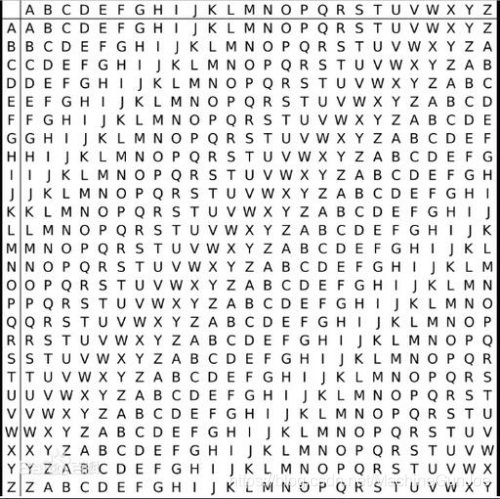24、培根密码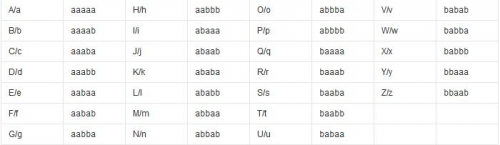25、猪圈密码。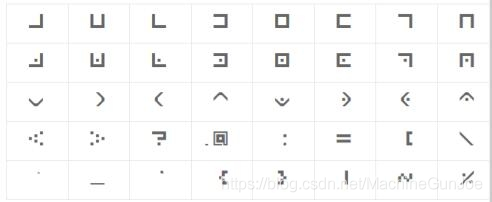猪圈密码在线解密平台：http://ctf.ssleye.com/pigpen.html

26、【字母表顺序】-数字  　　加密的时候，经常要把A~Z这26个字母转换成数字，最常见的一种方法就是取字母表中的数字序号。A代表1，B代表2，C代表3...

字母 A B C D E F G H I J K L M N O P Q R S T U V W X Y Z
数字 1 2 3 4 5 6 7 8 9 10 11 12 13 14 15 16 17 18 19 20 21 22 23 24 25 26

27、【进制转换密码】

例如二进制：1110 10101 1101 10 101 10010 1111 1110 101
转为十进制：14 21 13 2 5 18 15 14 5
对应字母表：number

28、【倒序】

加密时为经常要对字符进行倒序处理。如果让你按abcdef...的顺序背出字母表的每个字母会很容易，
但是如果是zyxwvu...的顺序那就很难背出来了。一个很熟悉的单词，如果按相反的顺序拼写，可能就会感到很陌生。

例如“love”字母倒过来拼就是“evol”。

具体加密时倒序有很多种方案，需要灵活运用。例如：

每个单词的倒序：siht si a tset - this is a test
整句的倒序：tset a si siht - this is a test
数字的倒序：02 50 91 02 - 20 05 19 20(test)

29、【间隔】

单词之间的间隔一般使用空格。在加密时常常要去掉空格，但有时某些字母或数字来替代空格也不失为一种好的加密方案。
错误空格位置也会起到很强的误导作用。

例如：t hi sis at est - this is a test

30、【字母频率】  　　频率分析法可以有效的破解单字母替换密码。

关于词频问题的密码，我在这里提供英文字母的出现频率给大家，其中数字全部是出现的百分比：
a 8.2 b 1.5 c 2.8 d 4.3
e 12.7 f 2.2 g 2.0 h 6.1
i 7.0 j 0.2 k 0.8 l 4.0
m 2.4 n 6.7 o 7.5 p 1.9
q 0.1 r 6.0 s 6.3 t 9.1
u 2.8 v 1.0 w 2.4 x 0.2
y 2.0 z 0.1

词频法其实就是计算各个字母在文章中的出现频率，然后大概猜测出明码表，最后验证自己的推算是否正确。
这种方法由于要统计字母出现频率，需要花费时间较长。参考《跳舞的小人》和《金甲虫》。

31、【Polybius密码(Polybius Cipher)】

也称棋盘密码，是利用波利比奥斯方阵(Polybius Square)进行加密的密码方式，产生于公元前两世纪的希腊，
相传是世界上最早的一种密码。

假设我们需要发送明文讯息 “Attack at once”， 用一套秘密混杂的字母表填满波利比奥斯方阵，像是这样：

A D F G X
A b t a l p
D d h o z k
F q f v s n
G g j c u x
X m r e w y

i和j视为同一个字，使字母数量符合 5 × 5 格。之所以选择这五个字母，是因为它们译成摩斯密码时不容易混淆，
可以降低传输错误的机率。使用这个方格，找出明文字母在这个方格的位置，再以那个字母所在的栏名称和列名称代替这个字母。
可将该讯息转换成处理过的分解形式。

明文：A T T A C K A T O N C E

A,D,F,G,X也可以用数字1,2,3,4,5来代替，这样密文就成了：

13 12 12 13 43 25 13 12 23 35 43 53

-------------------------------------------------------------------------

1918年，第一次世界大战将要结束时，法军截获了一份德军电报，电文中的所有单词都由A、D、F、G、X五个字母拼成，
A、D、F、G、X即Polybius方阵中的前5个字母。

明文：A T T A C K A T O N C E

下一步，利用一个移位密钥加密。假设密钥是“CARGO”，将之写在新格子的第一列。再将上一阶段的密码文一列一列写进新方格里。

C A R G O
_________
A F A D A
D A F G F
D X A F A
D D F F X
G F X F X

最后，密钥按照字母表顺序“ACGOR”排序，再按照此顺序依次抄下每个字母下面的整列讯息，形成新密文。如下：

在实际应用中，移位密钥通常有两打字符那么长，且分解密钥和移位密钥都是每天更换的。

在1918年6月，再加入一个字V扩充。变成以6×6格共36个字符加密。这使得所有英文字母（不再将I和J视为同一个字）以及数字0到9都可混合使用。
这次增改是因为以原来的加密法发送含有大量数字的简短信息有问题。

33、【希尔密码(Hill Cipher)】

希尔密码就是矩阵乘法密码，运用基本矩阵论原理的替换密码。每个字母当作26进制数字：A=0, B=1, C=2... 一串字母当成n维向量，
跟一个n×n的密钥矩阵相乘，再将得出的结果模26。希尔密码的优点是完全隐藏了字符的频率信息，弱点是容易被已知明文攻击击破。

加密
例如：密钥矩阵
1 3
0 2

明文：HI THERE

去空格，2个字母一组，根据字母表顺序换成矩阵数值如下，末尾的E为填充字元：

HI TH ER EE
8 20 5 5
9 8 18 5

HI 经过矩阵运算转换为 IS，具体算法参考下面的说明：

|1 3| 8 e1*8+3*9=35 MOD26=9 =I
|0 2| 9 e0*8+2*9=18 MOD26=18=S

用同样的方法把“HI THERE”转换为密文“IS RPGJTJ”，注意明文中的两个E分别变为密文中的G和T。

解密
解密时，必须先算出密钥的逆矩阵，然后再根据加密的过程做逆运算。

逆矩阵算法公式：
|A B| = 1/(AD-BC) * | D -B|
|C D| |-C A|

例如密钥矩阵=
|1 7|
|0 3|
因此
|1 7| 的逆矩阵为： 9 * |3 -7|
|0 3| |0 1|

假设密文为“FOAOESWO”

FO AO ES WO
6 1 5 23
15 15 19 15

9* |3 -7| | 6| = 9*(3*6-7*15)=-783 mod26 = 23=W
|0 1| |15| = 9*(0*6+1*15)= 135 mod26 = 5 =E

所以密文“FOAOESWO”的明文为“WEREDONE”

-------------------------------------------------------------------------

34、【Playfair密码(Playfair Cipher)】

Playfair将明文中的双字母组合作为一个单元对待，并将这些单元转换为双字母组合。
加密后的字符出现的频率在一定程度上被均匀化。

5*5变换矩阵(I或J视为同一字符)：

C I P H E
R A B D F
G K L M N
O Q S T U
V W X Y Z

加密规则：按成对字母加密

相同对中的字母加分隔符(如x)
ballon -> ba lx lo on
同行取右边：he->ec
同列取下边：dm->mt
其他取交叉：kt->mq od->tr

例如：ballon -> ba lx lo on -> db sp gs ug

35、【费娜姆密码】（密码：00110110010001001100100010000010110；密钥：study）

二战时德军使用过的一种密码，其实是利用了二进制的表示法来替代字母，有如下的表格作为基础：

A 1000001 B 1000010 C 1000011 D 1000100 E 1000101 F 1000110 G 1000111 H 1001000 I 1001001 J 1001010

K 1001011 L 1001100 M 1001101 N 1001110 O 1001111 P 1010000 Q 1010001 R 1010010 S 1010011 T 1010100

U 1010101 V 1010110 W 1010111 X 1011000 Y 1011001 Z 1011010

那么，比如我们要加密“Hello”，密钥用“study”，则以如下方式进行加密：

H E L L O = 1001000 1000101 1001100 1001100 1001111
S T U D Y = 1010011 1010100 1010101 1000100 1011001

加密原则：1+1=0，0+0=0，1+0=1

于是得密文：00110110010001001100100010000010110

那么解题目中的密文，需要遵循以下几个原则和步骤，

1，划分，即每七个数字为一组；

2，对应，找出密文每个字母对应的数字，再与上述数字对应；

3，转换，遵循上述加密原则，逆用即可；

4，解密，得出新的一组数字，对应字母，得出明文。

二、对称密码。展开全文古典密码
• 常见古典密码

千次阅读 2021-07-21 22:31:49
古典密码 文章目录古典密码前言1.Affine(仿射加密)2.Bacon(培根加密)3.Brainfuck4.Caesar(凯撒加密)5.Fence(栅栏加密)6.Fenham(费纳姆加密)7.Morse(摩斯密码)8.Pigen(猪圈加密)9.Vigenere(维吉尼亚加密) 前言 ...

前言

系统的学习了一下古典密码，这里大概整理一下主要的加密方式以及实现加解密的python代码。

1.Affine(仿射加密)

单码加密法的另一种形式称为仿射加密法（affine cipher）。在仿射加密法中，字母表的字母被赋予一个数字，例如a=0，b=1，c=2…z=25。仿射加密法的密钥为0-25直接的数字对。

代码：

# 乘法逆元
def multiplicative_inverse(key_a, a_z=26):
inv = 1
while True:
if (key_a * inv) % a_z == 1:
break
inv = inv + 1
return inv

# 加密
def encipher(key_a, key_b, txt):
result = ""
if judge_a(key_a) == 0:
result = "key_a必须是1, 3, 5, 7, 9, 11, 15, 17, 19, 21, 23, 25"
else:
if isinstance(key_b, int):
for i in txt.lower():
if i.isalpha():
temp = ((ord(i) - 97) * key_a + key_b) % 26 + 97
result = result + chr(temp)
else:
result = result + i
else:
result = "key_b必须为整数"
return result

# 解密
def decipher(key_a, key_b, cipher):
result = ""

if judge_a(key_a) == 0:
result = "key_a必须是1, 3, 5, 7, 9, 11, 15, 17, 19, 21, 23, 25"
else:
if isinstance(key_b, int):
a_inv = multiplicative_inverse(key_a)
for i in cipher.lower():
if i.isalpha():
temp = (((ord(i) - 97) - key_b) * a_inv) % 26 + 97
result = result + chr(temp)
else:
result = result + i
else:
result = "key_b必须为整数"
return result

def judge_a(key_a):
range_a = (1, 3, 5, 7, 9, 11, 15, 17, 19, 21, 23, 25)
if key_a in range_a:
return key_a
else:
return 0

if __name__ == "__main__":
a = 3
b = -1
text = 'hello word'
print(encipher(a, b, text))
print(decipher(a, b, 'ulggp npyi'))

输出：

ulggp npyi
hello word

2.Bacon(培根加密)

培根密码，又名倍康尼密码（英语：Bacon’s cipher）是由法兰西斯·培根发明的一种隐写术。
加密时，明文中的每个字母都会转换成一组五个英文字母。

'A': 'aaaaa', 'B': 'aaaab', 'C': 'aaaba', 'D': 'aaabb', 'E': 'aabaa', 'F': 'aabab',
'G': 'aabba',
'H': 'aabbb', 'I': 'abaaa', 'J': 'abaab', 'K': 'ababa', 'L': 'ababb', 'M': 'abbaa',
'N': 'abbab',
'O': 'abbba', 'P': 'abbbb', 'Q': 'baaaa', 'R': 'baaab', 'S': 'baaba', 'T': 'baabb',
'U': 'babaa', 'V': 'babab', 'W': 'babba', 'X': 'babbb', 'Y': 'bbaaa', 'Z': 'bbaab'

培根密码实际上就是一种替换密码，根据所给表一一对应转换即可加密解密 。它的特殊之处在于：可以通过不明显的特征来隐藏密码信息，比如大小写、正斜体等，只要两个不同的属性，密码即可隐藏。
解密时，将上述方法倒转。所有字体一转回A，字体二转回B，以后再按上表拼回字母。

代码：

def encipher1(txt):
bacon_dict1 = {'A': 'aaaaa', 'B': 'aaaab', 'C': 'aaaba', 'D': 'aaabb', 'E': 'aabaa', 'F': 'aabab', 'G': 'aabba',
'H': 'aabbb', 'I': 'abaaa', 'J': 'abaab', 'K': 'ababa', 'L': 'ababb', 'M': 'abbaa', 'N': 'abbab',
'O': 'abbba', 'P': 'abbbb', 'Q': 'baaaa', 'R': 'baaab', 'S': 'baaba', 'T': 'baabb',
'U': 'babaa', 'V': 'babab', 'W': 'babba', 'X': 'babbb', 'Y': 'bbaaa', 'Z': 'bbaab'}
result = ""
txt = txt.upper()
for i in txt:
try:
result = result + bacon_dict1[i]
except KeyError:
result = result + i
return result

def encipher2(txt):
bacon_dict2 = {'a': 'AAAAA', 'b': 'AAAAB', 'c': 'AAABA', 'd': 'AAABB', 'e': 'AABAA', 'f': 'AABAB', 'g': 'AABBA',
'h': 'AABBB', 'i': 'ABAAA', 'j': 'ABAAA', 'k': 'ABAAB', 'l': 'ABABA', 'm': 'ABABB', 'n': 'ABBAA',
'o': 'ABBAB', 'p': 'ABBBA', 'q': 'ABBBB', 'r': 'BAAAA', 's': 'BAAAB', 't': 'BAABA',
'u': 'BAABB', 'v': 'BAABB', 'w': 'BABAA', 'x': 'BABAB', 'y': 'BABBA', 'z': 'BABBB'}
result = ""
txt = txt.lower()
for i in txt:
try:
result = result + bacon_dict2[i]
except KeyError:
result = result + i
return result

# 文本->bacon
def encipher(txt):
results = [encipher1(txt), encipher2(txt)]
return results

def decipher1(txt):
bacon_dict1_txt = {'aaaaa': 'A', 'aaaab': 'B', 'aaaba': 'C', 'aaabb': 'D', 'aabaa': 'E', 'aabab': 'F', 'aabba': 'G',
'aabbb': 'H', 'abaaa': 'I', 'abaab': 'J', 'ababa': 'K', 'ababb': 'L', 'abbaa': 'M', 'abbab': 'N',
'abbba': 'O', 'abbbb': 'P', 'baaaa': 'Q', 'baaab': 'R', 'baaba': 'S', 'baabb': 'T',
'babaa': 'U', 'babab': 'V', 'babba': 'W', 'babbb': 'X', 'bbaaa': 'Y', 'bbaab': 'Z'}
result = ""
txt = txt.lower()
for i in range(0, len(txt), 5):
try:
result = result + bacon_dict1_txt[txt[i:i + 5]]
except KeyError:
result = result + "?"
return result

def decipher2(txt):
bacon_dict2_txt = {'AAAAA': 'a', 'AAAAB': 'b', 'AAABA': 'c', 'AAABB': 'd', 'AABAA': 'e', 'AABAB': 'f', 'AABBA': 'g',
'AABBB': 'h', 'ABAAA': '(i/j)', 'ABAAB': 'k', 'ABABA': 'l', 'ABABB': 'm', 'ABBAA': 'n',
'ABBAB': 'o', 'ABBBA': 'p', 'ABBBB': 'q', 'BAAAA': 'r', 'BAAAB': 's', 'BAABA': 't',
'BAABB': '(u/v)', 'BABAA': 'w', 'BABAB': 'x', 'BABBA': 'y', 'BABBB': 'z'}
result = ""
txt = txt.upper()
for i in range(0, len(txt), 5):
try:
result = result + bacon_dict2_txt[txt[i:i + 5]]
except KeyError:
result = result + '?'
return result

# bacon->文本
def decipher(txt):
results = [decipher1(txt), decipher2(txt)]
return results

if __name__ == '__main__':
print(encipher("sfauh"))
print(decipher("abbaabbaaa"))

输出：

ulggp npyi
hello word

3.Brainfuck

是一种极小化的计算机语言，它是由Urban Müller在1993年创建的。由于fuck在英语中是脏话，这种语言有时被称为brainf*ck或brainf** k，甚至被简称为BF.
这种语言，是一种按照“Turing complete（图灵完备）”思想设计的语言，它的主要设计思路是：用最小的概念实现一种“简单”的语言，BrainF**k 语言只有八种符号，所有的操作都由这八种符号的组合来完成。

代码：

def brain_fuck(code):
data = [0 for i in range(1000)]
pc = 0
ptr = 0
skip_loop = False
bracket_count = 0
stack = []
result = ''
while pc < len(code):
c = code[pc]
if skip_loop:
if c == '[':
bracket_count += 1
elif c == ']':
bracket_count -= 1
if bracket_count == 0:
skip_loop = False
pc += 1
continue
if c == '>':
ptr += 1
pc += 1
elif c == '<':
ptr -= 1
pc += 1
elif c == '+':
data[ptr] += 1
pc += 1
elif c == '-':
data[ptr] -= 1
pc += 1
elif c == '.':
result = result + chr(data[ptr])
pc += 1
elif c == ',':
pc += 1
elif c == '[':
if data[ptr] == 0:
# nonlocal bracket_count,skip_loop
bracket_count = 1
skip_loop = True
pc += 1
else:
pc += 1
stack.append(pc)
elif c == ']':
if data[ptr] == 0:
pc += 1
stack.pop()
else:
pc = stack[len(stack) - 1]
return result

if __name__ == '__main__':
print(brain_fuck('++++++++[>++++[>++>+++>+++>+<<<<-]>+>+>->>+[<]<-]>>.>---.+++++++..+++.>>.<-.<.+++.------.--------.>>+.>++.'))

输出：

Hello World!

4.Caesar(凯撒加密)

凯撒加密是古罗马恺撒大帝用来保护重要军情的加密系统。

“恺撒密码”它是一种替代密码，通过将字母按顺序推后3位起到加密作用，如将字母A换作字母D，将字母B换作字母E。据说恺撒是率先使用加密函的古代将领之一，因此这种加密方法被称为恺撒密码。

代码

# 判断密钥
def judge_key(k):
if 0 < k < 27 and isinstance(k, int):
return k
else:
return 0

def encipher(txt, k):
result = ""
if judge_key(k) == 0:
result = "密钥应为[1,26]的整数"
else:
result = result + str(k) + ':'

for i in txt:
if i.isupper():
result = result + chr(65 + (ord(i) - 65 + k) % 26)
elif i.islower():
result = result + chr(97 + (ord(i) - 97 + k) % 26)
else:
result = result + i
return result

# 返回所有偏移结果
def all_result(txt):
res = []
for n in range(1, 27):
res.append(encipher(txt, n))
return res

def rot13(txt):
res = "rot" + encipher(txt, 13)
return res

if __name__ == '__main__':
text=input("Please input the words : ")
results = all_result(text)
print(results)

输出：

Please input the words : Hello world
['1:Ifmmp xpsme', '2:Jgnnq yqtnf', '3:Khoor zruog', '4:Lipps asvph', '5:Mjqqt btwqi', '6:Nkrru cuxrj', '7:Olssv dvysk', '8:Pmttw ewztl', '9:Qnuux fxaum', '10:Rovvy gybvn', '11:Spwwz hzcwo', '12:Tqxxa iadxp', '13:Uryyb jbeyq',
'14:Vszzc kcfzr', '15:Wtaad ldgas', '16:Xubbe mehbt', '17:Yvccf nficu', '18:Zwddg ogjdv', '19:Axeeh phkew', '20:Byffi qilfx', '21:Czggj rjmgy', '22:Dahhk sknhz', '23:Ebiil tloia', '24:Fcjjm umpjb', '25:Gdkkn vnqkc', '26:Hello world']

5.Fence(栅栏加密)

所谓栅栏密码，就是把要加密的明文分成N个一组，然后把每组的第1个字连起来，形成一段无规律的话。 不过栅栏密码本身有一个潜规则，就是组成栅栏的字母一般不会太多。（一般不超过30个，也就是一、两句话）

代码：

# 栅栏范围
def get_field(txt):
field = []
for i in range(2, len(txt)):
if len(txt) % i == 0:
field.append(i)
return field

def encipher(txt, k):
field = get_field(txt)
txt_length = len(txt)
if k in field:
result = '' + str(k) + '栏:'
for m in range(k):
for n in range(m, txt_length, k):
result = result + txt[n]
else:
result = '密钥有误，应在范围'+str(field)
return result

# 输出所有可能的结果
def all_result(txt):
results = []
for k in get_field(txt):
results.append(encipher(txt, k))
return results

if __name__ == '__main__':
text = 'Helloworld'
print(encipher(text, 2))
print(all_result(text))

输出：

2栏:Hloolelwrd
['2栏:Hloolelwrd', '5栏:Hweolrllod']

6.Fenham(费纳姆加密)

费纳姆密码其实是一种由二进制产生的替换密码。

是双方约定一个数，明文加上这个数就是密文。这个数相当于密钥（可以是单词 词组 句子 几个字母也行）。

'A': '1000001', 'B': '1000010', 'C': '1000011', 'D': '1000100', 'E': '1000101', 'F': '1000110',
'G': '1000111', 'H': '1001000', 'I': '1001001', 'J': '1001010', 'K': '1001011', 'L': '1001100',
'M': '1001101', 'N': '1001110', 'O': '1001111', 'P': '1010000', 'Q': '1010001', 'R': '1010010',
'S': '1010011', 'T': '1010100', 'U': '1010101', 'V': '1010110', 'W': '1010111', 'X': '1011000',
'Y': '1011001', 'Z': '1011010'

例如：
明文： hello=1001000 1000101 1001100 1001100 1001111
密钥：crude=1000011 1010010 1010101 1000100 1000101
异或得到密文=0001011 0010111 0011001 0001000 0001010

代码：

char_num = {'A': '1000001', 'B': '1000010', 'C': '1000011', 'D': '1000100', 'E': '1000101', 'F': '1000110',
'G': '1000111', 'H': '1001000', 'I': '1001001', 'J': '1001010', 'K': '1001011', 'L': '1001100',
'M': '1001101', 'N': '1001110', 'O': '1001111', 'P': '1010000', 'Q': '1010001', 'R': '1010010',
'S': '1010011', 'T': '1010100', 'U': '1010101', 'V': '1010110', 'W': '1010111', 'X': '1011000',
'Y': '1011001', 'Z': '1011010'}

num_char = {'1000001': 'A', '1000010': 'B', '1000011': 'C', '1000100': 'D', '1000101': 'E', '1000110': 'F',
'1000111': 'G', '1001000': 'H', '1001001': 'I', '1001010': 'J', '1001011': 'K', '1001100': 'L',
'1001101': 'M', '1001110': 'N', '1001111': 'O', '1010000': 'P', '1010001': 'Q', '1010010': 'R',
'1010011': 'S', '1010100': 'T', '1010101': 'U', '1010110': 'V', '1010111': 'W', '1011000': 'X',
'1011001': 'Y', '1011010': 'Z'}

# 转换
def txt_num(txt):
txt = txt.upper()
result = ""
for i in txt:
result = result + char_num[i]
return result

# 最终数字转换为字母
def num_list(txt):
num = 0
s = []
while True:
s.append(txt[num:num + 7])
num += 7
if num > len(txt) - 7:
break
return s

# 加密 文本内容
def encipher(txt, k):
result = ''
if txt.isalpha() and k.isalpha():
txt = txt_num(txt)
k = txt_num(k)
j = len(k)
for i in range(0, len(txt)):
if txt[i] == k[i % j]:
result += '0'
else:
result += '1'
else:
result = "明文与密钥应为纯字母"
return result

# 解密 文本为数字格式
def decipher(txt, k):
result = ''
if k.isalpha():
k = txt_num(k)
j = len(k)
for i in range(0, len(txt)):
if txt[i] == k[i % j]:
result += '0'
else:
result += '1'
result = num_list(result)
result_char = ""
for i in result:
try:
result_char = result_char + num_char[i]
except KeyError:
result_char = result_char + '?'
result = result_char
else:
result = "密钥应为纯字母"
return result

if __name__ == '__main__':
# 加解密
text = '00010110010111001100100010000001010'
# 读文本文件
key = "crude"
text_2 = "hello"
print(encipher(text_2, key))
print(decipher(text, key))

输出:

00010110010111001100100010000001010
HELLO

7.Morse(摩斯密码)

摩尔斯电码是一种时通时断的信号代码，通过不同的排列顺序来表达不同的英文字母、数字和标点符号，是由美国人萨缪尔·摩尔斯在1836年发明。
每一个字符(字母或数字)对应不同的序列(由点和划组成)。
一般来说，任何一种能把书面字符用可变长度的信号表示的编码方式都可以称为摩尔斯电码。

'A': '.-', 'B': '-...', 'C': '-.-.', 'D': '-..', 'E': '.', 'F': '..-.', 'G': '--.',
'H': '....', 'I': '..', 'J': '.---', 'K': '-.-', 'L': '.-..', 'M': '--', 'N': '-.',
'O': '---', 'P': '.--．', 'Q': '--.-', 'R': '.-.', 'S': '...', 'T': '-',
'U': '..-', 'V': '...-', 'W': '.--', 'X': '-..-', 'Y': '-.--', 'Z': '--..',

'0': '-----', '1': '.----', '2': '..---', '3': '...--', '4': '....-',
'5': '.....', '6': '-....', '7': '--...', '8': '---..', '9': '----.',

'.': '.-.-.-', ':': '---...', ',': '--..--', ';': '-.-.-.', '?': '..--..',
'=': '-...-', "'": '.----.', '/': '-..-.', '!': '-.-.--', '-': '-....-',
'_': '..--.-', '"': '.-..-.', '(': '-.--.', ')': '-.--.-', '$': '...-..-', '@': '.--.-.', '+': '.-.-.' 代码： # "01"—>".-" def translate_string(txt): table = ''.maketrans('01', '.-') txt = txt.translate(table) return txt # 文本->摩斯密码 def encipher(txt): result = "" morse_dict = { 'A': '.-', 'B': '-...', 'C': '-.-.', 'D': '-..', 'E': '.', 'F': '..-.', 'G': '--.', 'H': '....', 'I': '..', 'J': '.---', 'K': '-.-', 'L': '.-..', 'M': '--', 'N': '-.', 'O': '---', 'P': '.--．', 'Q': '--.-', 'R': '.-.', 'S': '...', 'T': '-', 'U': '..-', 'V': '...-', 'W': '.--', 'X': '-..-', 'Y': '-.--', 'Z': '--..', '0': '-----', '1': '.----', '2': '..---', '3': '...--', '4': '....-', '5': '.....', '6': '-....', '7': '--...', '8': '---..', '9': '----.', '.': '.-.-.-', ':': '---...', ',': '--..--', ';': '-.-.-.', '?': '..--..', '=': '-...-', "'": '.----.', '/': '-..-.', '!': '-.-.--', '-': '-....-', '_': '..--.-', '"': '.-..-.', '(': '-.--.', ')': '-.--.-', '$': '...-..-',
'@': '.--.-.', '+': '.-.-.'
}
txt = txt.upper()
for i in txt:
try:
result = result + morse_dict[i] + '/'
except KeyError:
result = result + i + '/'
return result.strip('/')

# 摩斯密码—>文本
def decipher(txt, sign=" "):
result = ""
morse_dict = {
".-": "A", "-...": "B", "-.-.": "C", "-..": "D", ".": "E", "..-.": "F", "--.": "G",
"....": "H", "..": "I", ".---": "J", "-.-": "K", ".-..": "L", "--": "M", "-.": "N",
"---": "O", ".--．": "P", "--.-": "Q", ".-.": "R", "...": "S", "-": "T",
"..-": "U", "...-": "V", ".--": "W", "-..-": "X", "-.--": "Y", "--..": "Z",

"-----": "0", ".----": "1", "..---": "2", "...--": "3", "....-": "4",
".....": "5", "-....": "6", "--...": "7", "---..": "8", "----.": "9",

".-.-.-": ".", "---...": ":", "--..--": ",", "-.-.-.": ";", "..--..": "?",
"-...-": "=", ".----.": "'", "-..-.": "/", "-.-.--": "!", "-....-": "-",
"..--.-": "_", ".-..-.": '"', "-.--.": "(", "-.--.-": ")", "...-..-": "\$",
".--.-.": "@", ".-.-.": "+",
}

# "0/1"->"./-"
if '0' or '1' in txt:
txt = translate_string(txt)

# sign('/' or ' ')
if '/' in txt:
sign = '/'

# 分割，字符串string，分割标识符sign
lists = txt.split(sign)
for code in lists:
try:
result = result + morse_dict[code]
except KeyError:
result = result + "?"
return result

if __name__ == "__main__":
print(encipher("Hello world"))
print(decipher('...././.-../.-../---/ /.--/---/.-./.-../-..'))

输出：

...././.-../.-../---/ /.--/---/.-./.-../-..
HELLO?WORLD

8.Pigen(猪圈加密)

猪圈密码（亦称朱高密码、共济会暗号、共济会密码或共济会员密码），是一种以格子为基础的简单替代式密码。即使使用符号，也不会影响密码分析，亦可用在其它替代式的方法。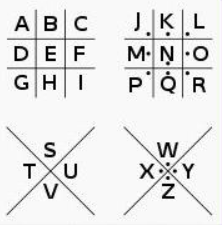代码：

def encipher(txt):
pigpen_dict = {'a': 'j', 'b': 'k', 'c': 'l', 'd': 'm', 'e': 'n', 'f': 'o', 'g': 'p', 'h': 'q', 'i': 'r',
'j': 'a', 'k': 'b', 'l': 'c', 'm': 'd', 'n': 'e', 'o': 'f', 'p': 'g', 'q': 'h', 'r': 'i',
's': 'w', 't': 'x', 'u': 'y', 'v': 'z', 'w': 's', 'x': 't', 'y': 'u', 'z': 'v'}
result = ""
txt = txt.lower()
for i in txt:
try:
result = result + pigpen_dict[i]
except KeyError:
result = result + i
return result

if __name__ == '__main__':
x = encipher("hello world")
print(x)

输出：

qnccf sficm

9.Vigenere(维吉尼亚加密)

维吉尼亚密码（又译维热纳尔密码）是使用一系列凯撒密码组成密码字母表的加密算法，属于多表密码的一种简单形式。
在一个凯撒密码中，字母表中的每一字母都会作一定的偏移，例如偏移量为3时，A就转换为了D、B转换为了E……而维吉尼亚密码则是由一些偏移量不同的恺撒密码组成。
为了生成密码，需要使用表格法。这一表格（如图1所示）包括了26行字母表，每一行都由前一行向左偏移一位得到。具体使用哪一行字母表进行编译是基于密钥进行的，在过程中会不断地变换。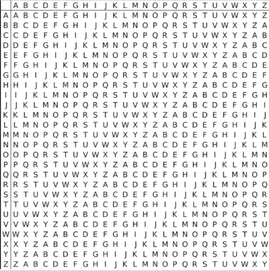例如，假设明文为：
ATTACKATDAWN
选择某一关键词并重复而得到密钥，如关键词为LEMON时，密钥为：
LEMONLEMONLE
对于明文的第一个字母A，对应密钥的第一个字母L，于是使用表格中L行字母表进行加密，得到密文第一个字母L。类似地，明文第二个字母为T，在表格中使用对应的E行进行加密，得到密文第二个字母X。以此类推，可以得到：
明文：ATTACKATDAWN
密钥：LEMONLEMONLE
密文：LXFOPVEFRNHR

代码：

letter_list = 'ABCDEFGHIJKLMNOPQRSTUVWXYZ'  # 字母表

# 根据输入的key生成key列表
def get_key_list(k):
k_list = []
for ch in k:
k_list.append(ord(ch.upper()) - 65)
return k_list

# 加密
def encipher(txt, k):
result = ""
k_list = get_key_list(k)
i = 0
for ch in txt:  # 遍历明文
if 0 == i % len(k_list):
i = 0
if ch.isalpha():  # 明文是否为字母,如果是,则判断大小写,分别进行加密
if ch.isupper():
result += letter_list[(ord(ch) - 65 + k_list[i]) % 26]
i += 1
else:
result += letter_list[(ord(ch) - 97 + k_list[i]) % 26].lower()
i += 1
else:  # 如果密文不为字母,直接添加到密文字符串里
result += ch
return result

# 解密
def decipher(txt, k):
result = ""
k_list = get_key_list(k)
i = 0
for ch in txt:  # 遍历密文
if 0 == i % len(k_list):
i = 0
if ch.isalpha():  # 密文为否为字母,如果是,则判断大小写,分别进行解密
if ch.isupper():
result += letter_list[(ord(ch) - 65 - k_list[i]) % 26]
i += 1
else:
result += letter_list[(ord(ch) - 97 - k_list[i]) % 26].lower()
i += 1
else:  # 如果密文不为字母,直接添加到明文字符串里
result += ch
return result

if __name__ == '__main__':
key = 'computer'
plaintext = "hello world"
ciphertext = encipher(plaintext, key)
print(ciphertext)
ciphertext = 'jsxai psinr'
plaintext = decipher(ciphertext, key)
print(plaintext)

输出：

jsxai psinr
hello world

展开全文python
• 古典密码技术Python 密码术：具有经典加密算法（例如Caesar密码，Hill密码和Vigenere密码）的Python程序。Python
• 古典密码的概念： 古典密码是密码学中的其中一个类型，其大部分加密方式都是利用【替换式密码】或【移项式密码】，有时则是两者的混合。 其于历史中经常使用，但现代已经很少使用，大部分的已经不再使用了。 一般而...
• 密码学，就是研究如何将一个内容（可以是字符串、文件、二进制流）通过一系列算法转换成另一种内容的学科。从广义上来看，有一个算法可以将字符串转换成另一个字符串即可，即该算法可逆不可逆都可以；从狭义上来看，...加密解密 数据安全 base64
• 古典密码及现代密码分组密码与流密码总结 古典密码大部分加密方式是利用替换式密码或移项式密码，有时是二者的混合。一般情况下，一种古典密码体制包含一个字母表，以及一个操作规则或一种操作设备，到了现代基本不...
• 古典密码实验报告.doc 哈尔滨工程大学实验报告实验名称古典密码算法班级学号姓名实验时间2014年4月成绩指导教师实验室名称哈尔滨工程大学实验室与资产管理处制一、实验名称古典密码算法2、实验目的通过编程实现经典...
• 涅普冬令营第四课------古典密码学介绍 入口 1.什么是密码学 密码学是一个多面的世界,对一些人来说,它是一个侦探与保密的世界,对另一些人来说,它是数学与计算机的世界。无论你如何看待它,密学都是神秘而富有冒险...python 加密解密
• 实验报告：通过编程实现替代密码算法和置换密码算法，算法描述，代码实现。
• 古典密码 1.单表密码（仿射加密） 仿射加密变化是：y=ax+b 算法： 仿射密码是一种表单代换密码，字母表的每个字母相应的值使用一个简单的数学函数对应一个数值，再把对应数值转换成字母。 A B C D E F G H I J K...
• 古代密码 数据的保密基于加密算法的保密。 Scytale密码 使用一条纸袋作为载体，环绕在一根固定半径的圆柱上。 加密 在绕好的纸带上写上明文。 解开缠绕后，就是加密好的、无序的密文。 圆柱的半径就是密钥。 ...
• 置换密码 古典密码算法

热门讨论 2011-11-21 23:08:48
置换密码 置换密码算法的原理是不改变明文字符，而是按照某一规则重新排列消息中的比特或字符顺序，才而实现明文信息的加密。置换密码有时又称为换位密码。 矩阵换位法是实现置换密码的一种常用方法。它将明文中的......